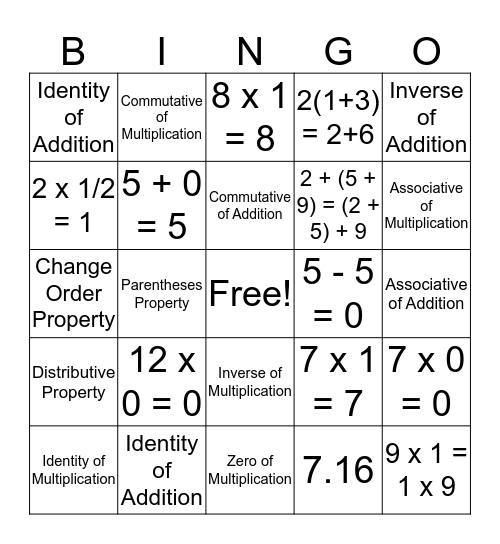# Number Properties BingoThis bingo card has a free space and 24 words: 12 x 0 = 0, Identity of Addition, Commutative of Addition, Identity of Multiplication, Inverse of Addition, Identity of Addition, 2 x 1/2 = 1, Commutative of Multiplication, 5 - 5 = 0, Inverse of Multiplication, 2 + (5 + 9) = (2 + 5) + 9, Change Order Property, 7 x 1 = 7, 7 x 0 = 0, 5 + 0 = 5, 7.16, Associative of Addition, 8 x 1 = 8, Associative of Multiplication, Parentheses Property, 9 x 1 = 1 x 9, Distributive Property, 2(1+3) = 2+6 and Zero of Multiplication.

⚠ This card has duplicate items: Identity of Addition (2)

## Play Online